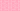PricingResources

# Discount factor table and definition

Written by

To calculate an investment’s net present value (NPV), you must first determine its discount factor. In other words, the discount factor measures the present value of an investment’s future worth. Find out what this means, how to calculate discount factor, and how it’s applied in finance below.

## What is the discount factor?

The discount factor formula offers a way to calculate the net present value (NPV). It’s a weighing term used in mathematics and economics, multiplying future income or losses to determine the precise factor by which the value is multiplied to get today’s net present value. This can be applied to goods, services, or investments, and is frequently used in corporate budgeting to determine whether a proposal will add future value.

Any discount factor equation uses the assumption that today’s money will be worth less in the future due to factors like inflation, which gives the discount factor a value between zero and one.

## Discount factor and net present value

The discount factor and discount rate are closely related, but while the discount rate looks at the current value of future cash flow, the discount factor applies to NPV. With these figures in hand, you can forecast an investment’s expected profits or losses, or its net future value.

## Calculations with the discount factor formula

As you can see from the breakdown above, there are multiple uses of the discount factor:

• To calculate net present value

• To assist with financial modelling

• To complete a discounted cash flow analysis

As a result, this handy little formula could be used by everyone from insurance companies to investors.

The general discount factor formula is:

To use this formula, you’ll need to find out the periodic interest rate or discount rate. This can easily be determined by dividing the annual discount factor interest rate by the total number of payments per year. You’ll also need to know the total number of payments that will be made.

## How to calculate discount factor

You can create a discount factor template or table in Excel to work out these calculations, by entering the formula above with your own figures. For example, a table might look like this:

 B C D E F 2 Period 1 2 3 4 3 Undiscounted Cash Flow \$100,000 \$100,000 \$100,000 \$100,000 4 Discount Factor Formula =1/1*(1+\$C\$4)^C2) =1/1*(1+\$C\$4)^D2) =1/1*(1+\$C\$4)^E2) =1/1*(1+\$C\$4)^F2) 5 Discount Factor 0.93 0.86 0.79 0.74

This shows the decreasing discount factor over time, whether it’s an annual discount factor or a shorter time frame to reflect your accounting period.

For example, to calculate discount factor for a cash flow one year in the future, you could simply divide 1 by the interest rate plus 1. For an interest rate of 5%, the discount factor would be 1 divided by 1.05, or 95%.

Once you have your discount factor and discount rate calculated, you can then use them to determine an investment’s net present value. Add together the present value of all positive cash flows, subtracting the present value of negative cash flows. Applying the interest rate, you’ll end up with the net present value. There are many discount factor calculators that will apply these formulas, or you can use Excel for an analysis.

## Discount factor benefits

Understanding the discount factor is helpful as it gives a visual representation of the impacts of compounding over time. This helps calculate discounted cash flow. As the discount rate grows over time, the cash flow decreases, making it a way to represent the time value of money in a decimal representation. Overall, investors can use this type of discount factor template to translate future investment returns into net present value.

## We can help

GoCardless helps you automate payment collection, cutting down on the amount of admin your team needs to deal with when chasing invoices. Find out how GoCardless can help you with ad hoc payments or recurring payments.